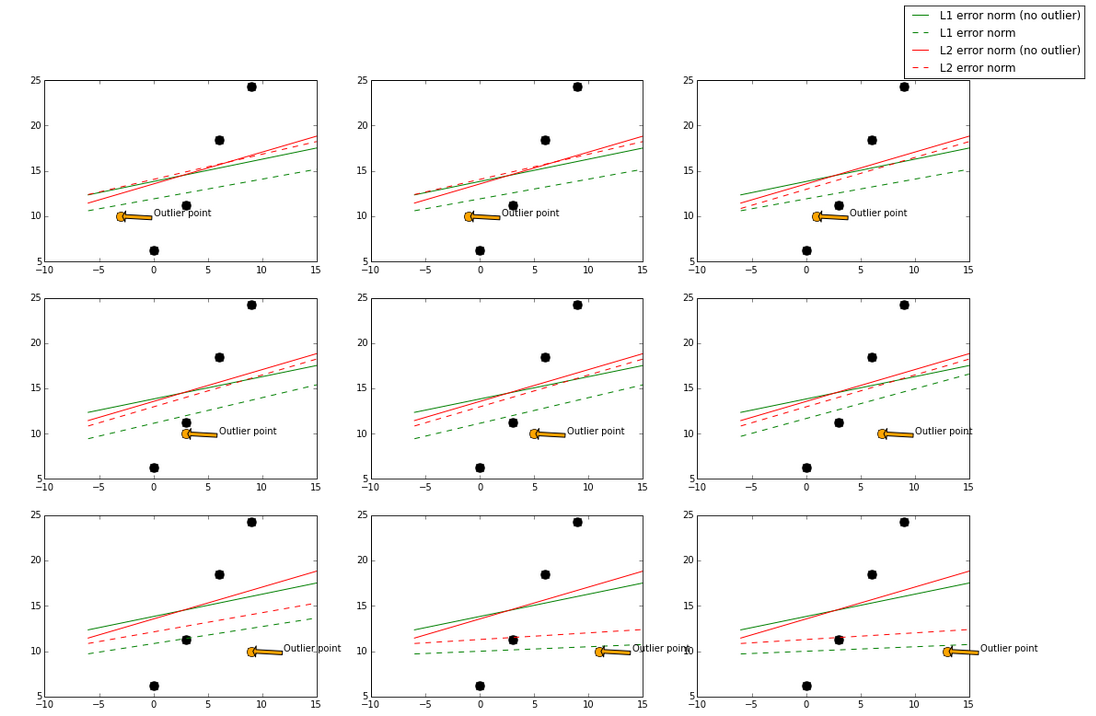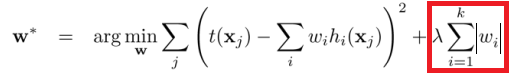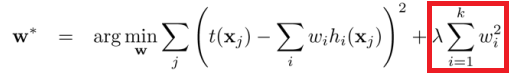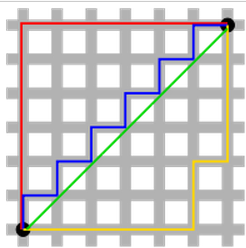# L1与L2损失函数和正则化的区别

2018/10/21 10:47

在机器学习实践中，你也许需要在神秘的L1和L2中做出选择。通常的两个决策为：1) L1范数 vs L2范数 的损失函数； 2) L1正则化 vs L2正则化。

### 作为损失函数

L1范数损失函数，也被称为最小绝对值偏差（LAD），最小绝对值误差（LAE）。总的说来，它是把目标值（$Y_{i}$）与估计值（$f(x_{i})$）的绝对差值的总和（$S$）最小化：

$$S=\sum\limits_{i=1}^{n}|Y_{i}-f(x_{i})|.$$

L2范数损失函数，也被称为最小平方误差（LSE）。总的来说，它是把目标值（$Y_{i}$）与估计值（$f(x_{i})$）的差值的平方和（$S$）最小化：

$$S=\sum\limits_{i=1}^{n}\Big(Y_{i}-f(x_{i})\Big)^{2}.$$

L1范数与L2范数作为损失函数的区别能快速地总结如下：

L2损失函数 L1损失函数

鲁棒性（robustness），根据维基百科，被解释为：

稳定性，根据维基百科，被解释为：### 作为正规化

在机器学习中，正规化是防止过拟合的一种重要技巧。从数学上讲，它会增加一个正则项，防止系数拟合得过好以至于过拟合。L1与L2的区别只在于，L2是权重的平方和，而L1就是权重的和。如下：L2正则化 L1正则化

解的唯一性是一个更简单的性质，但需要一点想象。首先，看下图：0
0 收藏

### 作者的其它热门文章0 评论
0 收藏
0